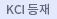#검색
다국어 입력

http://chineseinput.net/에서 pinyin(병음)방식으로 중국어를 변환할 수 있습니다.

변환된 중국어를 복사하여 사용하시면 됩니다.

예시)
• 中文 을 입력하시려면 zhongwen을 입력하시고 space를누르시면됩니다.
• 北京 을 입력하시려면 beijing을 입력하시고 space를 누르시면 됩니다.
닫기

검색결과 좁혀 보기

• 좁혀본 항목 보기순서

• 원문유무
• 음성지원유무
• 원문제공처
• 등재정보
• 학술지명
• 주제분류
• 발행연도
• 작성언어
• 저자

오늘 본 자료

• 오늘 본 자료가 없습니다.
더보기
• 무료
• 기관 내 무료
• 유료
••중금속이온이 시멘트의 수화 및 미세구조에 미치는 영향

The effect on the hydration of cement was that Cu and Pb reacted with alkali to form soluble hydrates at theinitial stage and then there followed a slow reaction forming insoluble metal hydroxides. These hydroxides were deposited on the surface of cement particles providing a barrier against further hydration. But as a slow reaction continued, the insoluble layers were eventually destroyed and the hydration reaction resumed. Thereafter, another retardation occured by restricting the polymerization of silicates, shown by FT-IR spectroscopy analysis. In the case of Cr, as its reaction with cement caused H2O, the coordinator of Cr complex, to replace or polymerize with OH-, the formation of Cr complex promoted the leakage of OH- and increased the heat of dissolution. So the total heat evolution during hydration was larger than that in the case of Pb or Cu. The retarding effect of heavy metal ions was in the order Pb>Cu>Cr.

•3CaO.$Al_2O_3$의 수화반응에 미치는 글루콘산나트륨읨 영향(I) -흡착거동-

The adsorption behavior of 3CaO.$Al_2O_3$-sodium gluconate-$H_2O$ system by measuring adsorp-tion isotherm DTA and IR sepctra. The adsorbed amount of sodium gluconate on 3CaO.$Al_2O_3$ is exceedingly larger than 3CaO.$SiO_2$ and portland cement. From the DAT experiment the formation of complex is observed by the characteristic exothermic peak of the complex at 45$0^{\circ}C$ It is now strong deduced that the chemical bonding between gluconate anion and 3CaO.$Al_2O_3$ should be coordinative due to the complex formation on the surface 3CaO.$Al_2O_3$ from the IR spectra of sod-ium gluconate only and 3CaO.$Al_2O_3$ -sodium gluconate-$H_2O$.

•Supercritical Drying에 의한 $TiO_2-SiO_2$ 이성분계 Aerogel의 제조 및 그 특성분석

TiO2-SiO2 homogeneous sol was prepared by means of complexing titanium iso-propoxide with acetylacetone. Crack-free, monolithic binary TiO2-SiO2 aerogels were obtained by evacuating the solvent under the pressure of 2,200 psi at 28$0^{\circ}C$, which was determined as a supercritical condition. The microstructure of the binary aerogels was observed to be finer and more homogeneous as the Ti content decreased. The microstructure was related to the optical transition of aerogels from opaque to transluscent.

•알콕사이드로부터 Seed가 첨가된 알루미나의 제조(I): 분말특성

The powder characteristics of seeded alumina prepared from alkoxide by sol-gel method were studied. When ${\alpha}$-Al2O3 seeded powders used, these ${\alpha}$ phase transformation temperatures decreased than those of unseeded powders by 110$^{\circ}C$ and fine powders under 0.1 $\mu\textrm{m}$ could be obtained. When Fe-nitrate added powders used, fast transformation rate resulted from ionic effects of Fe3+, but hard aggregated morphology exhibited. When ${\alpha}$-Al2O3 and Fe nitrate simultaneously added, these powders represented lower transformation temperature but resulted in microstructure with aggregated particles.

•알콕사이드로부터 Seed가 첨가된 알루미나의 제조: (II) 소결특성

The sintering behaviors of seeded alumina from alkoxide were studied. Room temp. desiccated powder exhibited better sintering behavior due to its packing ability and powder activity. $\alpha$-Al2O3 2wt% seeded compacts sintered with 97.5%TD, 1~3${\mu}{\textrm}{m}$ diameters at 140$0^{\circ}C$, 2hrs. Fe-nitrate doped compacts resulted in enhanced initial sintering behavior due to ionic effects of Fe3+ but failed to refined microstructure.

•알콕사이드로부터 알루미나 미분말의 합성과 그 소결특성

The powder characterization and sintering behavior of alkoxide-derived alumina powders prepared by esterification were investigated. The dried powders were calcined at 700$^{\circ}C$, 900$^{\circ}C$, 1080$^{\circ}C$, 1170$^{\circ}C$ for 1 h. and the resulting crystalline forms were amorphous, {{{{ gamma }} phase, {{{{ theta }}+{{{{ alpha }} phase, and {{{{ alpha }} phase, respectively. The sinterability was best in the case of being calcined at 1170$^{\circ}C$. It was most effective to disperse sintering additive in the initial stage from which acetate was formed. At 1600$^{\circ}C$ for 4 h, 98.5% of relative density was obtained when MgO and Fe2O3 were simultaneously doped, and dense sintered body whose density was near theoretical value was obtained when heating schedule was controlled.

• Fuzzy Delphi 法을 이용한 일반지수 예측 전문가 시스템 구축

전문가 내지 구성원의 주관적인 의견에 의존하는 방법의 하나인 델파이법(Delphi Method)은 관련자료가 불충분한 중·장기 예측, 전략결정 등에 이용되고 있다. 이 방법은 더욱 발전시킨 퍼지 델파이법(FuzzyDelphi Method)은 델파이법에 퍼지숫자(Fuzzy Number)의 개념을 도입하여 정확한 예측을 하고자 하는 것이다. 또한 이러한 예측치가 삼각 퍼지 숫자(Triangular Fuzzy Number)로 주어져 불확실성에 대한 예측과 의견종합을 쉽게 하며 전문가에 의해 추정된 삼각 퍼지 숫자의 입력을 통해 그 추정치들의 비유사도(Dissemblance Index)와 퍼지거리(Fuzzy Distance)를 계산하고 간단한 그래프를 다시 전문가에게 피드백(Feedback)할 수 있도록 나타내어지는 과정을 Code화하여 전문가들로 하여금 다양한 정보를 통하여 좀 더 정확한 추정치를 예측하고자 한다.

•Al-Sec-Butoxide의 가수분해시에 있어서 $\alpha$-$Al_2O_3$종의 동시첨가에 의한 열적 전이거동

The thermal behavior of transformation and characteristics of seeded sample powders prepared by simultaneous $\alpha$-Al2O3 seed addition with water on the Al-sec-butoxide hydrolysis were studied. $\alpha$-Al2O3 seed particles are shown to act as nuclei for transformation of $\theta$-to $\alpha$-Al2O3 and to result in an increase in thetransformation kinetics and lowering of the transformation temperature by as much as 143$^{\circ}C$. Simultaneous seed addition on the hydrolysis resulted in uniform dispersin and creation of nucleation site on seed surface and only 0.1wt% seeding lowered the transformation temperature by as much as 115$^{\circ}C$. For 3wt% seed addition, $\alpha$-Al2O3 single phase was obtained at 95$0^{\circ}C$ for 100 minutes and the specific surface area of products were lowered to 11.9$m^2$/g as compared with that of $\alpha$-Al2O3 powder prepared without seed at 115$0^{\circ}C$ ; 15.1$m^2$/g due to depression of vermicular structure growth.